Last updated 06-27-2014

This tutorial is a joint product of the Statnet Development Team:

Martina Morris (University of Washington)
Mark S. Handcock (University of California, Los Angeles)
Carter T. Butts (University of California, Irvine)
David R. Hunter (Penn State University)
Steven M. Goodreau (University of Washington)
Skye Bender de-Moll (Oakland)
Pavel N. Krivitsky (University of Wollongong)

For general questions and comments, please refer to statnet users group and mailing list
http://statnet.csde.washington.edu/statnet_users_group.shtml

## 1. Getting Started

Open an R session, and set your working directory to the location where you would like to save this work.

To install all of the packages in the statnet suite:

install.packages('statnet')
library(statnet)

Or, to only install the specific statnet packages needed for this tutorial:

install.packages('tergm') # will install the network package
install.packages('sna')

After the first time, to update the packages one can either repeat the commands above, or use:

update.packages('name.of.package')

For this tutorial, we will need one additional package (coda), which is recommended (but not required) by ergm:

install.packages('coda')

Make sure the packages are attached:

library(statnet)

or

library(tergm)
library(sna)
library(coda)

Check package version

# latest versions:  tergm 3.1.4, ergm 3.1.2, network 1.9.0, networkDynamic 0.6.3 (as of 6/27/2014)
sessionInfo()

Set seed for simulations – this is not necessary, but it ensures that we all get the same results (if we execute the same commands in the same order).

set.seed(0)

## 2. A quick review of static ERGMs

Exponential-family random graph models (ERGMs) represent a general class of models based in exponential-family theory for specifying the probability distribution underlying a set of random graphs or networks. Within this framework, one can—among other tasks—obtain maximum-likehood estimates for the parameters of a specified model for a given data set; simulate additional networks with the underlying probability distribution implied by that model; test individual models for goodness-of-fit, and perform various types of model comparison.

The basic expression for the ERGM class can be written as:

$$P(Y=y)=\frac{\exp(\theta'g(y))}{k(\theta)}$$

where:

• Y is the random variable for the state of the network (with realization y)

• $$g(y)$$ is the vector of model statistics for network y,

• $$\theta$$ is the vector of coefficients for those statistics

• $$k(\theta)$$ represents the quantity in the numerator summed over all possible networks (typically constrained to be all networks with the same node set as y).

This can be re-expressed in terms of the conditional log-odds of a single actor pair:

$$\operatorname{logit}{P(Y_{ij}=1 \mid y^{c}_{ij}) = \ln{\frac{P(Y_{ij}=1 \mid y^{c}_{ij})}{P(Y_{ij}=0 \mid y^{c}_{ij})}} = \theta'\delta(g(y))_{ij}}$$

where:

• $$Y_{ij}$$ is the random variable for the state of the actor pair $$i,j$$ (with realization $$y_{ij}$$)

• $$y^{c}_{ij}$$ signifies the complement of $$y_{ij}$$, i.e. all dyads in the network other than $$y_{ij}$$.

• $$\delta(g(y))_{ij}$$ equals $$g(y^{+}_{ij})-g(y^{-}_{ij})$$, where

• $$y^{+}_{ij}$$ is defined as $$y^{c}_{ij}$$ along with $$y_{ij}$$ set to 1

• $$y^{-}_{ij}$$ is defined as $$y^{c}_{ij}$$ along with $$y_{ij}$$ set to 0.

• That is, $$\delta(g(y))_{ij}$$ equals the value of $$g(y)$$ when $$y_{ij}=1$$ minus the value of $$g(y)$$ when $$y_{ij}=0$$, but all other dyads are as in $$g(y)$$. This emphasizes the log-odds of an individual tie conditional on all others.

• We call $$g(y)$$ the statistics of the model, and $$\delta(g(y))_{ij}$$ the change statistics for actor pair $$y_{ij}$$.

Fitting an ERGM usually begins with obtaining data:

data("florentine")
ls()
plot(flobusiness)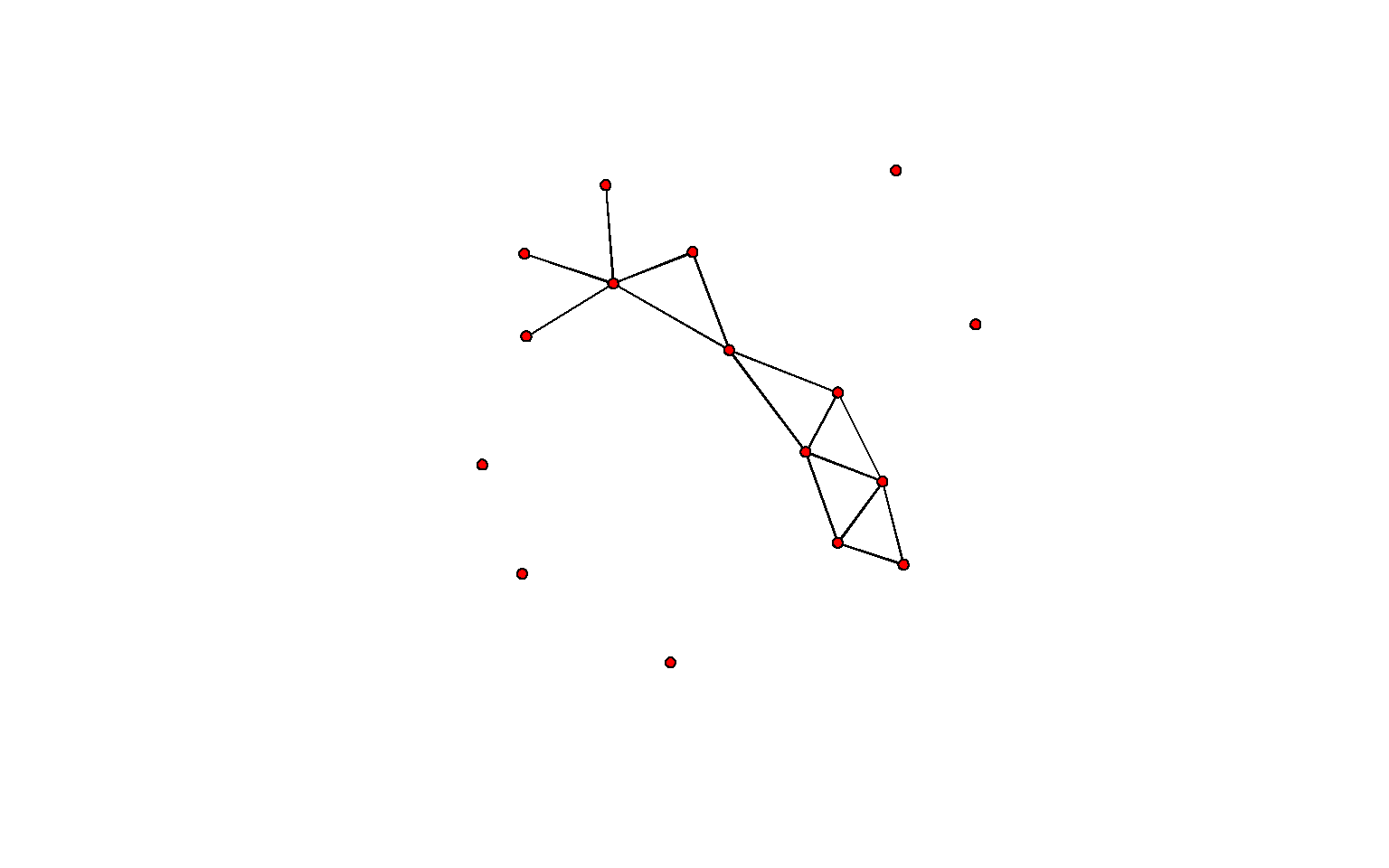To refresh our memories on ERGM syntax, let us fit a cross-sectional example. Just by looking at the plot of flobusiness, we might guess that there are more triangles than expected by chance for a network of this size and density, and thus that there is some sort of explicit triangle closure effect going on. One useful way to model this effect in ERGMs that has been explored in the literature is with a gwesp statistic. When the alpha parameter of this statistic equals 0, then the statistic counts the number of edges in the network that are in at least one triangle. You can see this by summarizing this network and then counting them for yourself in the visualization:

summary(flobusiness~edges+gwesp(0, fixed=T))
        edges gwesp.fixed.0
15            12 
fit1 <- ergm(flobusiness~edges+gwesp(0,fixed=T))
Iteration 1 of at most 20:
Convergence test P-value: 2.5e-275
The log-likelihood improved by 0.08012
Iteration 2 of at most 20:
Convergence test P-value: 1.1e-44
The log-likelihood improved by 0.01473
Iteration 3 of at most 20:
Convergence test P-value: 7.7e-09
The log-likelihood improved by 0.003271
Iteration 4 of at most 20:
Convergence test P-value: 7e-01
Convergence detected. Stopping.
The log-likelihood improved by < 0.0001

This model was fit using MCMC.  To examine model diagnostics and check for degeneracy, use the mcmc.diagnostics() function.
summary(fit1)

==========================
Summary of model fit
==========================

Formula:   flobusiness ~ edges + gwesp(0, fixed = T)

Iterations:  20

Monte Carlo MLE Results:
Estimate Std. Error MCMC % p-value
edges           -3.354      0.606      0  <1e-04 ***
gwesp.fixed.0    1.551      0.577      0  0.0082 **
---
Signif. codes:  0 '***' 0.001 '**' 0.01 '*' 0.05 '.' 0.1 ' ' 1

Null Deviance: 166.4  on 120  degrees of freedom
Residual Deviance:  78.1  on 118  degrees of freedom

AIC: 82.1    BIC: 87.7    (Smaller is better.) 

How to interpret the coefficients? Conditional on the rest of the network, a given edge’s log-odds of existing depends on how much its presence changes the number of edges in at least one triangle in the network.

• If the answer is 0 (i.e. the two incident nodes have no relational partners in common), the log-odds are $$-3.354$$.
• If the answer is 1 then the log-odds are $$-3.354 + 1.551 = -1.803$$.
• Is the answer is 2 then the log-odds are $$-3.354 + 1.551{\times}2 = -0.252$$.
• The corresponding probabilities are $$3.4\%$$, $$14.1\%$$, and $$43.7\%$$.
• How can adding one edge change the number of edges in at least one triangle by 2?

With the estimation in place, we can simulate a new network from the given model:

sim1 <- simulate(fit1,nsim=1,
control=control.simulate.ergm(MCMC.burnin=1000))
plot(sim1)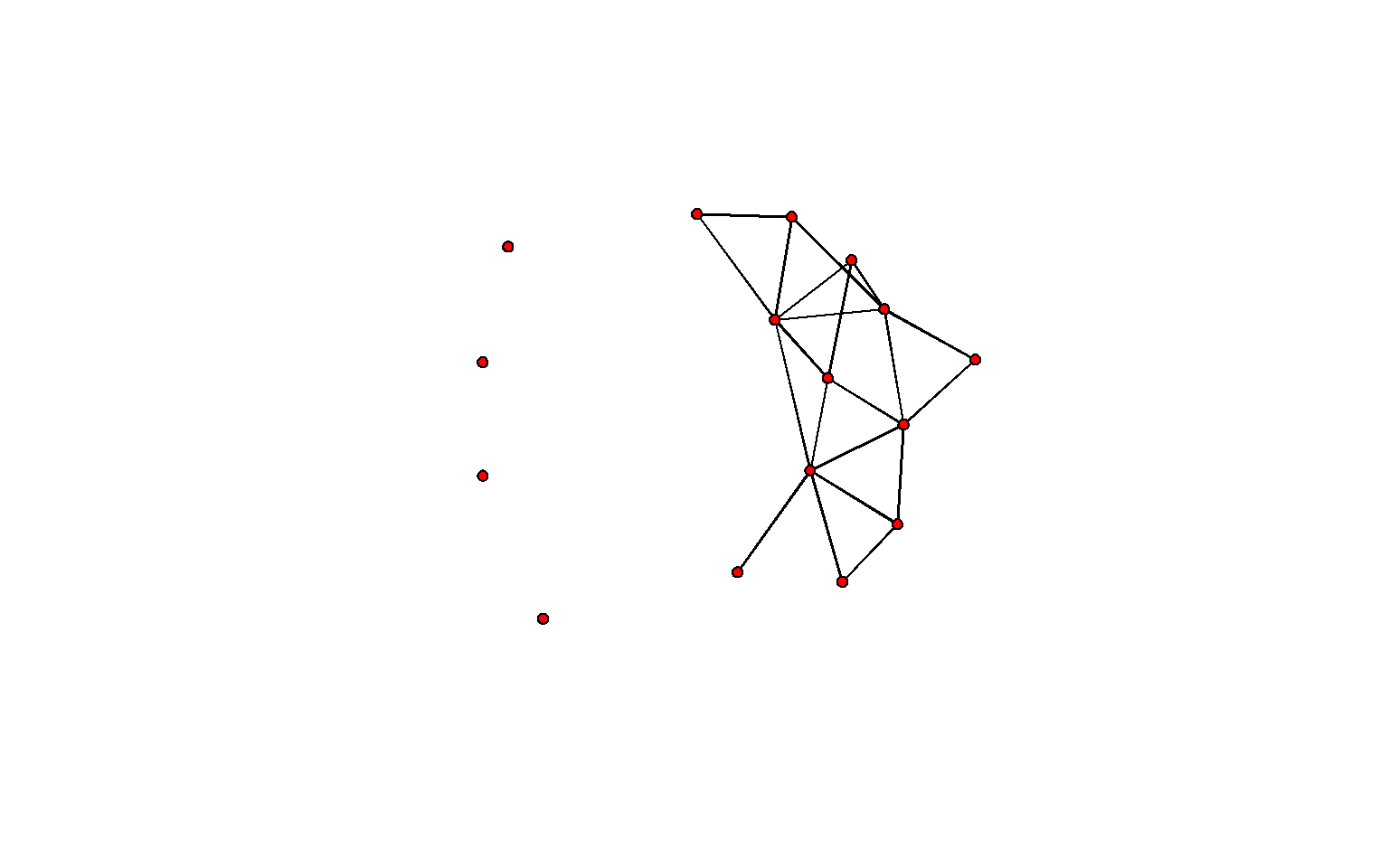## 3. An Introduction to STERGMs (non-technical)

• Separable Temporal ERGMs (STERGMs) are an extension of ERGMs for modeling dynamic networks in discrete time, introduced in Krivitsky and Handcock (2010).

• The cross-sectional ERGM entails a single network, and a single model on that network. STERGMs, in contrast, posit two models: one ERGM underlying relational formation, and a second one underlying relational dissolution. Specifying a STERGM thus entails writing two ERGM formulas instead of one.

• STERGMs also requires dynamic data, of course; such data can come in many forms, and we will cover a few examples today.

• This approach is not simply a methodological development, but a theoretical one as well, and one which matches common sense for many social processes. Think of romantic relations. It seems intuitive that the statistical model underlying relational formation (i.e. affecting who becomes partners with whom, out of the set of people who aren’t already) is likely to be different than the model underlying relational dissolution (i.e. affecting who breaks up with whom, out of the set of people currently in relationships). Any reasonable model of the former would need to include a variety of homophily parameters (mixing on age, for example). The latter may or may not. (Conditional on being in a relationship, does your difference in age affect your probability of breaking up? Perhaps, but probably not as fundametally or as strongly as for formation).

• In thinking about how STERGMs work, and how they relate to different data forms, it can be helpful to think of the approximation commonly used in epidemiology to consider basic disease dynamics:

$$prevalence = incidence x duration$$

• To translate this to dynamic networks: first think of a Bernoulli model where all ties are equally likely. This expression indicates that the number of ties present in the cross-section (prevalence) is a function of the rate at which they form (incidence) and the rate at which they dissolve (1/duration). The faster ties form, the more ties that are present in the cross-section. And the slower ties dissolve, the more ties that are present in the cross-section.

• This same concept extends to more elaborate models beyond just Bernoulli. Imagine a model with triangles in it—the faster that triangles are formed and the slower that they’re dissolved, the more that will be present in the cross-section.

• Using the notation from the ERGM review above: the two equations governing a STERGM are commonly called the formation equation and the dissolution (or persistence) equation, respectively:

$$\ln{\frac{P(Y_{ij,t+1}=1 \mid y^{c}_{ij}, Y_{ij,t}=0)}{P(Y_{ij,t+1}=0\mid y^{c}_{ij}, Y_{ij,t}=0)}} = \theta^+ \delta(g^+(y))_{ij}$$

$$\ln{\frac{P(Y_{ij,t+1}=1 \mid y^{c}_{ij}, Y_{ij,t}=1)}{P(Y_{ij,t+1}=0\mid y^{c}_{ij}, Y_{ij,t}=1)}} = \theta^- \delta(g^-(y))_{ij}$$

• These largely parallel the one ERGM equation. We have simply:

• added a time index to the tie values

• added in a new conditional. In the formation equation, the expression is conditional on the tie not existing at the prior time step, and in the dissolution equation it is conditional on the tie existing; and

• defined two $$\theta$$ and $$g$$ vectors instead of just one. Now there are $$\theta^+$$ and $$g^+(y)_{ij}$$, reflecting the coefficients and statistics in the formation model, and $$\theta^-$$ and $$g^-(y)_{ij}$$ reflecting those in the dissolution model.

• Notice that for the dissolution model, the $$P(Y_{ij,t+1}=1)$$ is in the numerator and the $$P(Y_{ij,t+1}=0)$$ is in the denominator. This is needed to make the mathematics parallel to that of the formation model; however, it is also what causes the model to be an expression of relational persistence rather than of dissolution. Of course, the probability of dissolution is merely 1 - persistence, so the model captures both phenomena, and conceptually we often think about relational dissolution in parallel with formation.

• To understand the implications of the two $$\theta$$ and $$g$$ vectors, imagine a model for romantic relationships that captures propensities for:

• people who are currently in one relationship to not form a new relationship as often as single people do; and

• those few people who are in two ongoing relationships to dissolve one of them more often than others do.

• Both the formation and dissolution (persistence) equations would contain the ergm term “concurrent”, which counts the number of nodes in the network of degree 2 or more.

• In both models, the coefficient for the concurrent term would be negative; new ties are relatively unlikely to form if they will generate a new node of degree 2 or more. And they are relatively unlikely to persist if they maintain the current number of nodes of degree 2 or more, when they could bring it down by dissolving.

• Finally, remember that the s in stergm stands for separable; this refers to the fact that the two models assume that relational formation and dissolution occur independently of one another within a time step. We explore how this works and its implications in the examples.

## 4. An Introduction to STERGMs (more formal)

To understand STERGMs formally, we first review the ERGM framework for cross-sectional or static networks again, this time with more complete notation. Let $$\mathbb{Y}\subseteq \{1,\dotsc,n\}^2$$ be the set of potential relations (dyads) among $$n$$ nodes, ordered for directed networks and unordered for undirected. We can represent a network $$\mathbf{y}$$ as a set of ties, with the set of possible sets of ties, $$\mathcal{Y}\subseteq 2^\mathbb{Y}$$, being the sample space: $$\mathbf{y} \in \mathcal{Y}$$. Let $$\mathbf{y}_{ij}$$ be 1 if $$(i,j)\in\mathbf{y}$$ — a relation of interest exists from $$i$$ to $$j$$ — and 0 otherwise.

The network also has an associated covariate array $$\mathbf{X}$$ containing attributes of the nodes, the dyads, or both. An exponential-family random graph model (ERGM) represents the pmf of $$\mathbf{Y}$$ as a function of a $$p$$-vector of network statistics $$g(\mathbf{Y},\mathbf{X})$$, with parameters $$\theta \in \mathbb{R}^p$$, as follows:

$$\mbox{Pr}\left(\mathbf{Y} = \mathbf{y} \mid \mathbf{X}\right) = \frac{\exp\left\{\theta\cdot g(\mathbf{y}, \mathbf{X})\right\} } {k(\theta, \mathbf{X}, \mathcal{Y})},$$

where the normalizing constant $$k(\theta, \mathbf{X}, \mathcal{Y}) = \sum\limits_{\mathbf{y}' \in \mathcal{Y}}\exp\left\{\theta\cdot g(\mathbf{y}', \mathbf{X})\right\}$$ is a summation over the space of possible networks on $$n$$ nodes, $$\mathcal{Y}$$. Where $$\mathcal{Y}$$ and $$\mathbf{X}$$ are held constant, as in a typical cross-sectional model, they may be suppressed in the notation. Here, on the other hand, the dependence on $$\mathcal{Y}$$ and $$\mathbf{X}$$ is made explicit.

In modeling the transition from a network $$\mathbf{Y}^{t}$$ at time $$t$$ to a network $$\mathbf{Y}^{t+1}$$ at time $$t+1$$, the separable temporal ERGM assumes that the formation and dissolution of ties occur independently from each other within each time step, with each half of the process modeled as an ERGM. For two networks (sets of ties) $$\mathbf{y},\mathbf{y}'\in \mathcal{Y}$$, let $$\mathbf{y} \supseteq \mathbf{y}'$$ if any tie present in $$\mathbf{y}'$$ is also present in $$\mathbf{y}$$. Define $$\mathcal{Y}^+(\mathbf{y})=\{\mathbf{y}'\in\mathcal{Y}:\mathbf{y}'\supseteq\mathbf{y}\}$$, the networks that can be constructed by forming ties in $$\mathbf{y}$$; and $$\mathcal{Y}^-(\mathbf{y})=\{\mathbf{y}'\in\mathcal{Y}:\mathbf{y}'\subseteq\mathbf{y}\}$$, the networks that can be constructed dissolving ties in $$\mathbf{y}$$.

Given $$\mathbf{y}^{t}$$, a formation network $$\mathbf{Y}^+$$ is generated from an ERGM controlled by a $$p$$-vector of formation parameters $$\theta^+$$ and formation statistics $$g^+(\mathbf{y}^+, \mathbf{X})$$, conditional on only adding ties:

$$\Pr\left(\mathbf{Y}^+ = \mathbf{y}^+\mid \mathbf{Y}^{t};\theta^+\right) = \frac{\exp\left\{\theta^+\cdot g^+(\mathbf{y}^+, \mathbf{X})\right\} } {k\left(\theta^+, \mathbf{X}, \mathcal{Y}^+(\mathbf{Y}^{t})\right)}, \quad \mathbf{y}^+ \in \mathcal{Y}^+(\mathbf{y}^{t})$$.

A dissolution network $$\mathbf{Y}^-$$ is simultaneously generated from an ERGM controlled by a (possibly different) $$q$$-vector of dissolution parameters $$\theta^-$$ and corresponding statistics $$g^-(\mathbf{y}^-, \mathbf{X})$$, conditional on only dissolving ties from $$\mathbf{y}^{t}$$, as follows:

$$\Pr\left(\mathbf{Y}^- = \mathbf{y}^-\mid \mathbf{Y}^{t};\theta^-\right) = \frac{\exp\left\{\theta^-\cdot g^-(\mathbf{y}^-, \mathbf{X})\right\} } {k\left(\theta^-, \mathbf{X}, \mathcal{Y}^-(\mathbf{Y}^{t})\right)}, \quad \mathbf{y}^- \in \mathcal{Y}^-(\mathbf{y}^{t}).$$

The cross-sectional network at time $$t+1$$ is then constructed by applying the changes in $$\mathbf{Y}^+$$ and $$\mathbf{Y}^-$$ to $$\mathbf{y}^{t}$$, as follows: $$\mathbf{Y}^{t+1} = \mathbf{Y}^{t}\cup (\mathbf{Y}^+ - \mathbf{Y}^{t}) \, - \, ( \mathbf{Y}^{t} - \mathbf{Y}^-).$$ which simplifies to either: $$\mathbf{Y}^{t+1} = \mathbf{Y}^+ - (\mathbf{Y}^{t} - \mathbf{Y}^-)$$ $$\mathbf{Y}^{t+1} = \mathbf{Y}^-\cup (\mathbf{Y}^+ - \mathbf{Y}^{t})$$

Visually, we can sum this up as: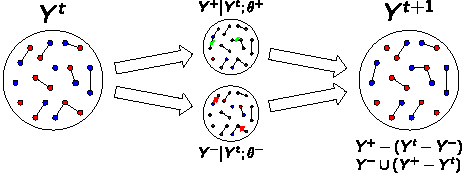## 5. Notes on model specification and syntax

Within statnet, an ERGM involves one network and one set of network statistics, so these are specified together using R’s formula notation:

my.network $$\sim$$ my.vector.of.model.terms

For a call to stergm, there is still one network (or a list of networks), but two formulas. These are now passed as three separate arguments: the network(s) (argument nw), the formation formula (argument formation), and the dissolution formula (argument dissolution). The latter two both take the form of a one-sided formula. E.g.:

        stergm(my.network,
formation= ~edges+kstar(2),
dissolution= ~edges+triangle
)

There are other features of a call to either ergm or stergm that will be important; we list these features here, and illustrate them in one or more examples during the workshop.

• To fix the coefficient for a particular network statistic, one uses offset notation.
For instance, to fix a dissolution model with only an edges term with parameter value 4.2, the dissolution formula would be:
dissolution = ~offset(edges)

and the corresponding argument for passing the parameter value would be:

offset.coef.diss = 4.2

• In parallel with ergm, any information used to specify the nature of the fitting algorithm is passed by specifying a vector called control.stergm to the control argument. For example:
control=control.stergm(MCMC.burnin=10000)

For a list of options, type ?control.stergm

• Another argument that the user must supply is estimate, which controls the estimation method. Unlike with cross-sectional ERGMs, there is not necessarily an obvious default here, as different scenarios are best fit with different approaches. The most important for the new user to recognize are EGMME (equilibrium generalized method of moments estimation) and CMLE (conditional maximum likelihood estimation). A good rule of thumb is that when fitting to two (or more) networks (i.e. with panel data), one should use estimate="CMLE"; and when fitting to a single cross-section with some duration information, use estimate="EGMME".

## 6. Example 1: Multiple network cross-sections

One common form of data for modeling dynamic network processes consists of observations of network structure at two or more points in time on the same node set. Many classic network studies were of this type, and data of this form continue to be collected and analyzed.

Let us consider the famous Sampson monastery data:

data(samplk)
ls()
 "fit1"        "flobusiness" "flomarriage" "metadata"    "samplk1"
 "samplk2"     "samplk3"     "sim1"       

To pass them into stergm, we need to combine them into a list:

samp <- list()
samp[] <- samplk1
samp[] <- samplk2
samp[] <- samplk3

Now we must decide on a model to fit to them. Plotting one network:

plot(samplk1)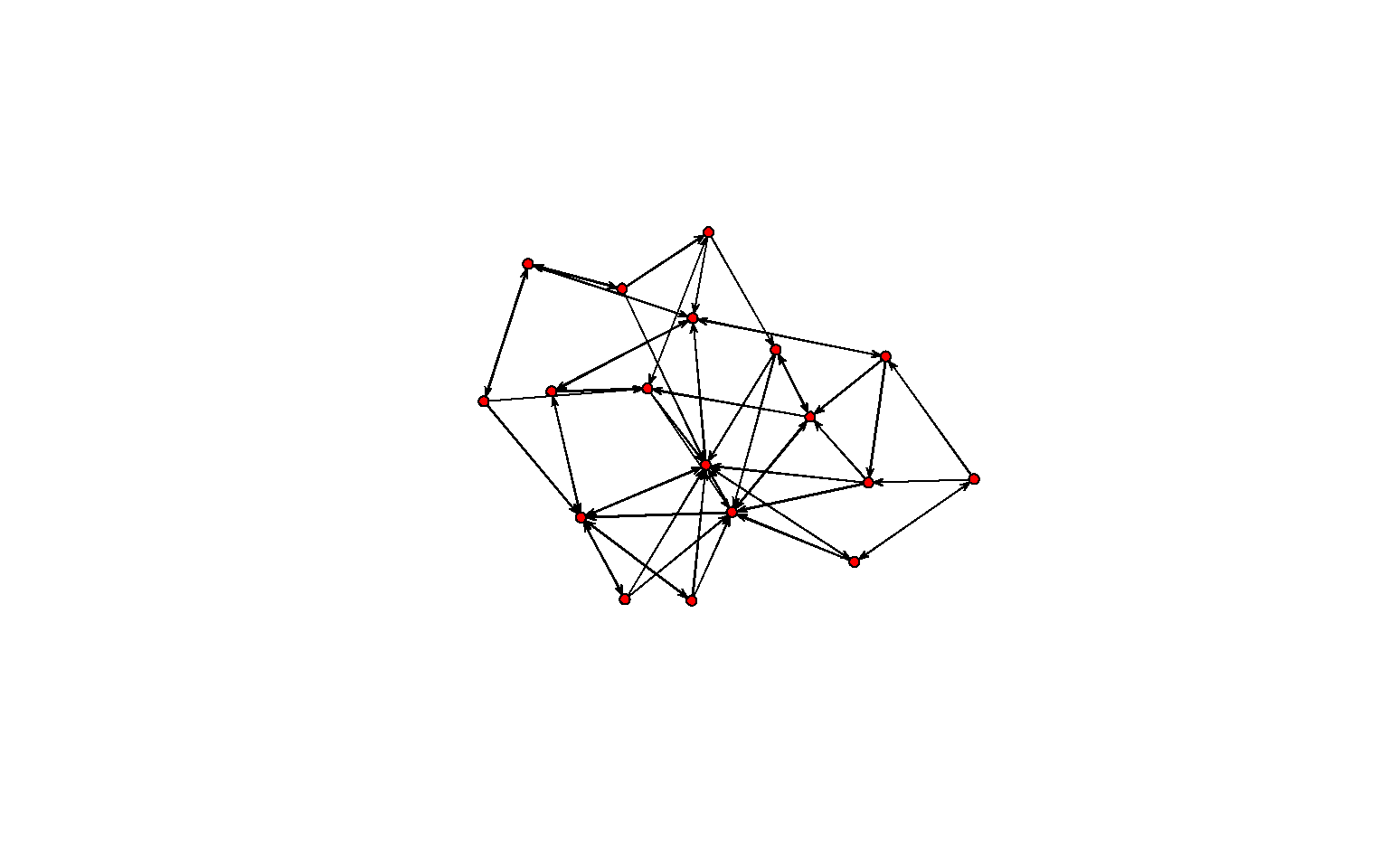we might get the idea to consider mutuality as a predictor of a directed edge. Also, since this is a directed network, and there appear to be a considerable number of triadic relations, it might be worth investigating the role of cyclicity vs. transitivity in the network. Although there are a number of ways to model this latter question, we will select the relatively robust measures transitiveties and cyclicalties (the number of ties $$i{\rightarrow}j$$ that have at least one two-path from $$i{\rightarrow}j$$ and from $$j{\rightarrow}i$$, respectively).

Since we have multiple network snapshots, and we have separate formation and dissolution models, we can estimate the degree to which closing a mutual dyad or closing a triad of each type predicts the creation of a tie, and also estimate the degree to which maintaining a mutual dyad or maintaining a triad of each type predicts the persistence of an existing tie. We might see different phenomena at work in each case; or the same phenomena, but with different coefficients.

In the following code, we pass five arguments: the series of networks that we want to model (samp), the formation formula, the dissolution formula, the fitting algorithm (in this case, conditional MLE is best since we have a network time series), and the list of time slices we wish to model (which includes all in the passed network series by default):

samp.fit <- stergm(samp,
formation= ~edges+mutual+cyclicalties+transitiveties,
dissolution = ~edges+mutual+cyclicalties+transitiveties,
estimate = "CMLE",
times=1:3
)
Fitting formation:
Iteration 1 of at most 20:

====== Lots of output snipped ======

This model was fit using MCMC.  To examine model diagnostics and check for degeneracy, use the mcmc.diagnostics() function.

And the results:

summary(samp.fit)

==============================
Summary of formation model fit
==============================

Formula:   ~edges + mutual + cyclicalties + transitiveties

Iterations:  20

Monte Carlo MLE Results:
Estimate Std. Error MCMC % p-value
edges            -3.468      0.337      0  <1e-04 ***
mutual            2.044      0.404      0  <1e-04 ***
cyclicalties     -0.138      0.200      0   0.490
transitiveties    0.393      0.235      0   0.094 .
---
Signif. codes:  0 '***' 0.001 '**' 0.01 '*' 0.05 '.' 0.1 ' ' 1

Null Deviance: 693  on 500  degrees of freedom
Residual Deviance: 240  on 496  degrees of freedom

AIC: 248    BIC: 265    (Smaller is better.)

================================
Summary of dissolution model fit
================================

Formula:   ~edges + mutual + cyclicalties + transitiveties

Iterations:  20

Monte Carlo MLE Results:
Estimate Std. Error MCMC % p-value
edges             0.207      0.301      0   0.492
mutual            0.797      0.513      0   0.123
cyclicalties     -0.192      0.251      0   0.447
transitiveties    0.507      0.282      0   0.075 .
---
Signif. codes:  0 '***' 0.001 '**' 0.01 '*' 0.05 '.' 0.1 ' ' 1

Null Deviance: 155  on 112  degrees of freedom
Residual Deviance: 137  on 108  degrees of freedom

AIC: 145    BIC: 156    (Smaller is better.) 

So, all else being equal, a relationship is much more likely to form if it will close a mutual pair; the log-odds are increased by $$2.044$$. Note that the most that the change statistic can equal in this case is 1.

The effect on persistence is also positive, but less strong and clear; the point estimate is an increase of $$0.797$$ in the log-odds of persistence.

Transitivity may also matter, perhaps slightly more so in terms of dissolution than formation.

If one wishes to include only a subset of times from one’s network series, one can alter the times argument:

samp.fit.2 <- stergm(samp,
formation= ~edges+mutual+cyclicalties+transitiveties,
dissolution = ~edges+mutual+cyclicalties+transitiveties,
estimate = "CMLE",
times=1:2
)

How do these coefficients compare? How about the standard errors?

## 7. Example 2: One network cross section and durational information

Now, let us imagine a different scenario in which we have observed two types of data: a single cross-sectional network, and a mean relational duration. Let us say the cross-sectional network is flobusiness, and the mean relational duration we have witnessed is 10 time steps. Furthermore, we are willing (for reasons of theory or convenience) to assume a purely homogeneous dissolution process (that is, every existing relationship has the same probability of dissolving as all others, and at all times).

For a cross-sectional ERGM, a purely homogeneous model is one with just a single term in it for an edge count. The same is true for either of the two formulas in a STERGM.

First, we specify formation and dissolution models (formation and dissolution). We will begin by assuming a formation model identical to the model we fit in the cross-sectional case:

formation = ~edges+gwesp(0,fixed=T)

Analogously to cross-sectional ERGMs, our assumption of completely homogeneous dissolution corresponds to a model with only an edgecount term in it. As we will see in the next step, however, we are going to supply the stergm alogrithm with the coefficient for our dissolution model, and not habve the algorithm try to estimate it. In STERGM notation this is:

dissolution = ~offset(edges)

Second, we calculate theta.diss. Our dissolution model is applied to the set of ties that exist at any given time point, as reflected in the conditional present in both the numerator and denominator:

$$\ln{\frac{P(Y_{ij,t+1}=1 \mid Y_{ij,t}=1)}{P(Y_{ij,t+1}=0\mid Y_{ij,t}=1)}} = \theta'\delta(g(y))_{ij}$$

Recall that the numerator represents the case where a tie persists to the next step, and the denominator represents the case where it dissolves.

Furthermore, the one term in our $$\delta(g(y))_{ij}$$ vector is the change statistic on network edge count, which equals one for all $$i,j$$.

We define the probability of persistance from one time step to the next for actor pair $$i,j$$ as $$p_{ij}$$, and the probability of dissolution as $$q_{ij}=1-p_{ij}$$. Our dissolution model is Bernoulli; that is, all edges have the same probability of dissolution, and thus of persistence, so we further define $$p_{ij}=p$$ for all $$i,j$$ and $$q_{ij}=q$$ for all $$i,j$$. Then:

$$\ln{(\frac{p_{ij}}{1-p_{ij}})}=\theta ' \delta(g(y))_{ij}$$

$$\ln{(\frac{p}{1-p})}=\theta$$

$$\ln{(\frac{1-q}{q})}=\theta$$

$$\ln{(\frac{1}{q}-1)}=\theta$$

And because this is a discrete memoryless process, durations are geometric; and for the geometric distribution, the mean is equal to the reciprocal of the dissolution probability. Symbolizing mean relational duration as $$d$$, we have $$d = \frac{1}{q}$$, and thus:

$$\theta = \ln{(d-1)}$$

So, for our dissolution model, theta.diss = $$\ln{(10-1)}$$ = $$\ln{9}$$ = $$2.197$$:

theta.diss <- log(9)

In short, because our dissolution model is dyadic independent, we can calculate it using a (rather simple) closed form solution.

Third, we decide upon our targets. For cross-sectional ERGMs, the model is by default fit using the sufficient statistics present in the cross-sectional network. For the STERGM with two cross-sections that we fit above, we also wanted to use the sufficient statistics present in the two observed networks. In the case of one cross-section but a formation and dissolution model, the reasonable default is less clear. Thus, the user is required to supply this information via the targets argument. This can take a one-sided formula listing the terms to be fit using the data in the network cross-section; or, if the formula is identical to either the formation or dissolution model, the user can simply pass the string "formation" or "dissolution", respectively. If one is specifying targets="formation", dissolution should be an offset, and vice versa. If the values to be targeted for those terms are anything other than the sufficient statistics present in the cross-sectional network, then those values can be passed with the argument target.stats. In this case, we want to use the sufficient statistics present in the cross-sectional network for the model terms in the formation model.

Finally, we estimate the formation model, conditional on the dissolution model. We put it all together for our first call to stergm, adding in one additional control argument that helps immensely with monitoring model convergence (and is just plain cool): plotting the progress of the coefficient estimates and the simulated sufficient statistics in real time. When one is using a GUI tool like RStudio, it helps to output this plotting to a separate window, which we do below with the function X11 (and then turn off that window again with dev.off()):

X11()
formation= ~edges+gwesp(0,fixed=T),
dissolution = ~offset(edges),
targets="formation",
offset.coef.diss = theta.diss,
estimate = "EGMME",
control=control.stergm(SA.plot.progress=TRUE)
)
dev.off()
Iteration 1 of at most 20:

====== Lots of output snipped ======

== Phase 3: Simulate from the fit and estimate standard errors.==

The real-time feedback suggests that the fitting went well, but let’s double-check:

mcmc.diagnostics(stergm.fit.1)
==========================
EGMME diagnostics
==========================
====== Lots of output snipped ======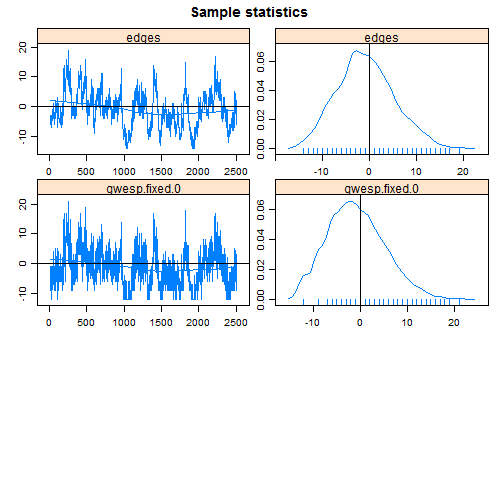Since these look good, we can next query the object in a variety of ways to see what we have:

stergm.fit.1
Formation Coefficients:
edges  gwesp.fixed.0
-6.46           2.29
Dissolution Coefficients:
edges
2.2  
names(stergm.fit.1)
  "network"         "formation"       "dissolution"
 "targets"         "target.stats"    "estimate"
 "covar"           "opt.history"     "sample"
 "sample.obs"      "control"         "reference"
 "mc.se"           "constraints"     "formation.fit"
 "dissolution.fit"
stergm.fit.1$formation ~edges + gwesp(0, fixed = T) stergm.fit.1$formation.fit

EGMME Coefficients:
edges  gwesp.fixed.0
-6.46           2.29  
summary(stergm.fit.1)

==============================
Summary of formation model fit
==============================

Formula:   ~edges + gwesp(0, fixed = T)

Iterations:  NA

Equilibrium Generalized Method of Moments Results:
Estimate Std. Error MCMC % p-value
edges            -6.46       1.77      0 0.00041 ***
gwesp.fixed.0     2.29       1.33      0 0.08658 .
---
Signif. codes:  0 '***' 0.001 '**' 0.01 '*' 0.05 '.' 0.1 ' ' 1

================================
Summary of dissolution model fit
================================

Formula:   ~offset(edges)

Iterations:  NA

Equilibrium Generalized Method of Moments Results:
Estimate Std. Error MCMC % p-value
edges      2.2         NA     NA      NA

The following terms are fixed by offset and are not estimated:
edges 

We have now obtained estimates for the coefficients of a formation model that, conditional on the stated dissolution model, yields simulated targets that matched those observed. Something very useful we have also gained in the process is the ability to simulate networks with the desired cross-sectional structure and mean relational duration. This ability serves us well for any application areas that requires us to simulate phenomena on dynamic networks, whether they entail the diffusion of information or disease, or some other process.

stergm.sim.1 <- simulate.stergm(stergm.fit.1, nsim=1,
time.slices = 1000)

Understanding this object requires us to learn about an additional piece of statnet functionality: the networkDynamic package.

## 8. networkDynamic

In statnet, cross-sectional networks are stored using objects of class network.
Tools to create, edit, and query network objects are in the package network. Dynamic networks are now stored as objects with two classes (network and networkDynamic). They can thus be edited or queried using standard functions from the network package, or using additional functions tailored specifically to the case of dynamic networks in the package networkDynamic.

To illustrate, let us begin with the network that we just created:

stergm.sim.1
NetworkDynamic properties:
distinct change times: 946
maximal time range: -Inf to Inf

Includes optional net.obs.period attribute:
Network observation period info:
Number of observation spells: 2
Maximal range of observations: 0 to 1001
Temporal mode: discrete
Time unit: step
Suggested time increment: 1

Network attributes:
vertices = 16
directed = FALSE
hyper = FALSE
loops = FALSE
multiple = FALSE
bipartite = FALSE
net.obs.period: (not shown)
total edges= 120
missing edges= 0
non-missing edges= 120

Vertex attribute names:
priorates totalties vertex.names wealth

Edge attribute names:
active 

We can deduce from the number of edges that this likely represents the cumulative network—that is, the union of all edges that exist at any point in time over the course of the simulation. This is because the total number of dyads in the network is (16 choose 2), or 120.

What does the network look like at different time points? The function network.collapse allows us to pull out the network at an instantaneous time point (with the argument at), while the function network.extract can pull out a given spell (with the arguments onset and terminus).

net500 <- network.collapse(stergm.sim.1,at=500)
net500
 Network attributes:
vertices = 16
directed = FALSE
hyper = FALSE
loops = FALSE
multiple = FALSE
bipartite = FALSE
total edges= 11
missing edges= 0
non-missing edges= 11

Vertex attribute names:
priorates totalties vertex.names wealth

No edge attributes

And we can look at the network structure:

plot(net500)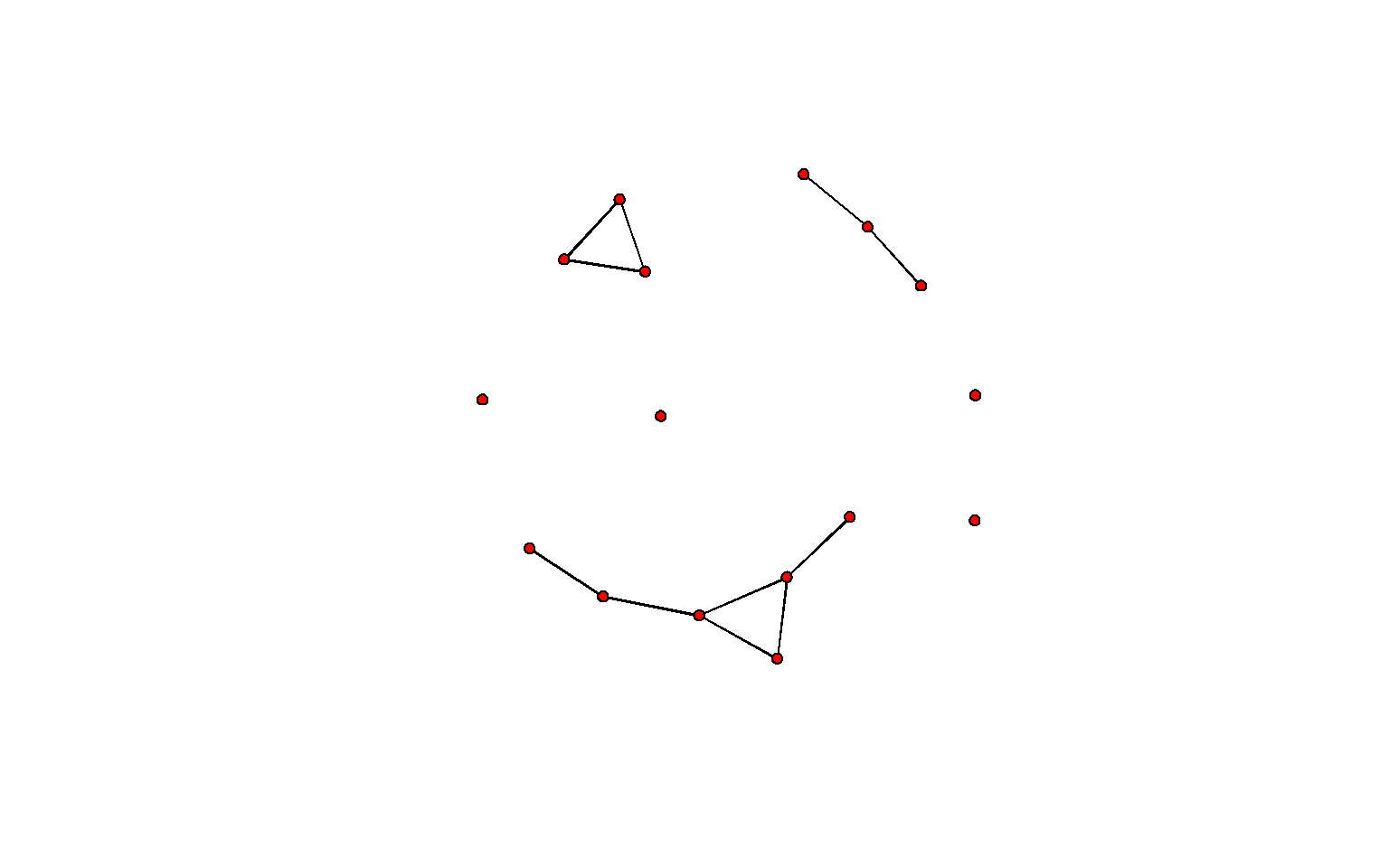How well do the cross-sectional networks within our simulated dynamic network fit the probability distribution implied by our model? We can check by considering the summary statistics for our observed network, and those for our cross-sectional networks. This is easy, since by default, the simulate command for a stergm object not only simulates a dynamic network, but calculates the statistics from the model for each time point. These are stored in attribute(simulated.networkDynamic)$stats. summary(flobusiness~edges+gwesp(0,fixed=T))  edges gwesp.fixed.0 15 12  colMeans(attributes(stergm.sim.1)$stats)
        edges gwesp.fixed.0
16.15         13.06 

And we can also easily look at a time series and histogram for each statistic:

plot(attributes(stergm.sim.1)$stats)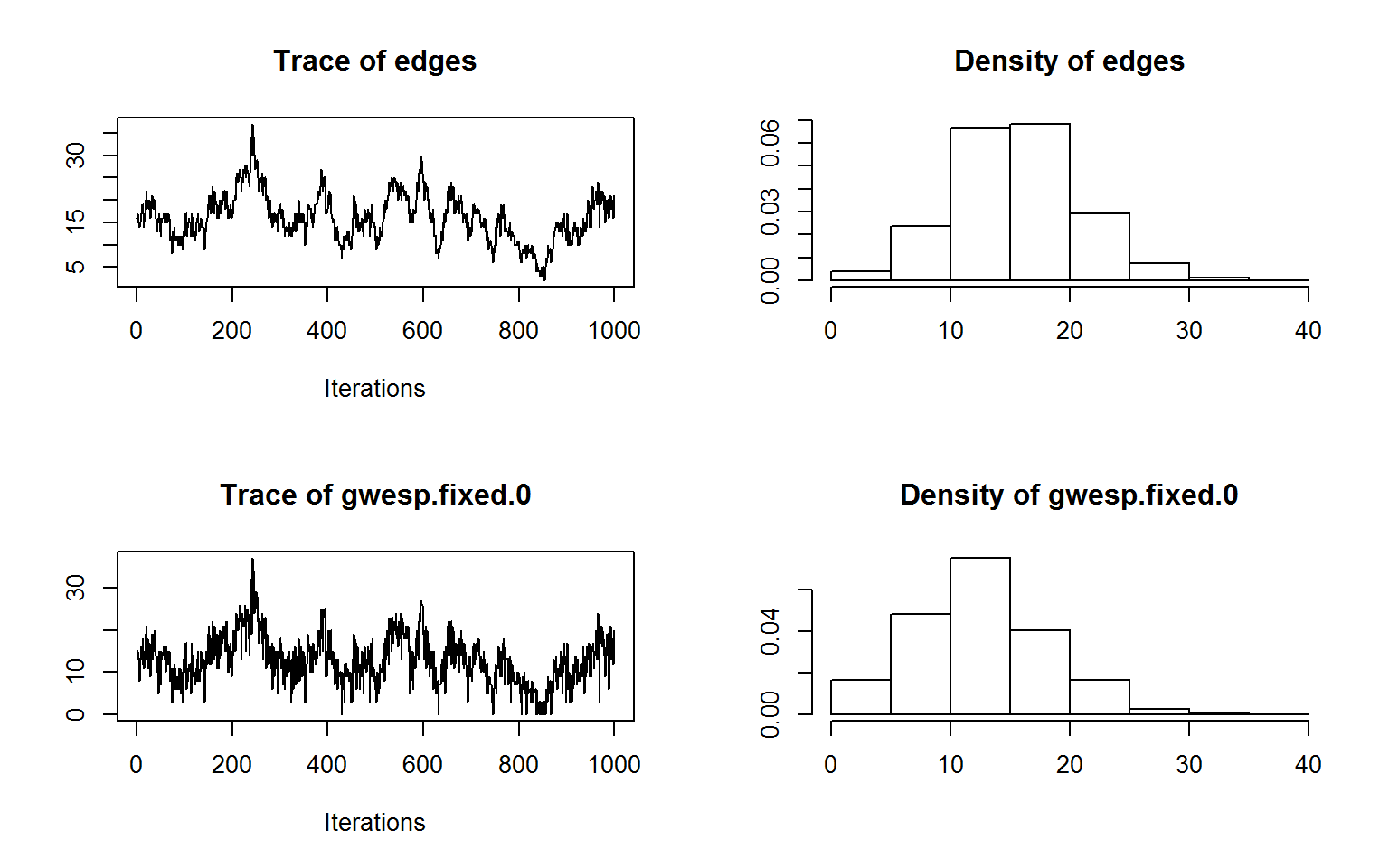Or even at a scatter plot of the two network statistics for each simulated network cross-section, to see how these co-vary for this model: plot(as.matrix(attributes(stergm.sim.1)$stats))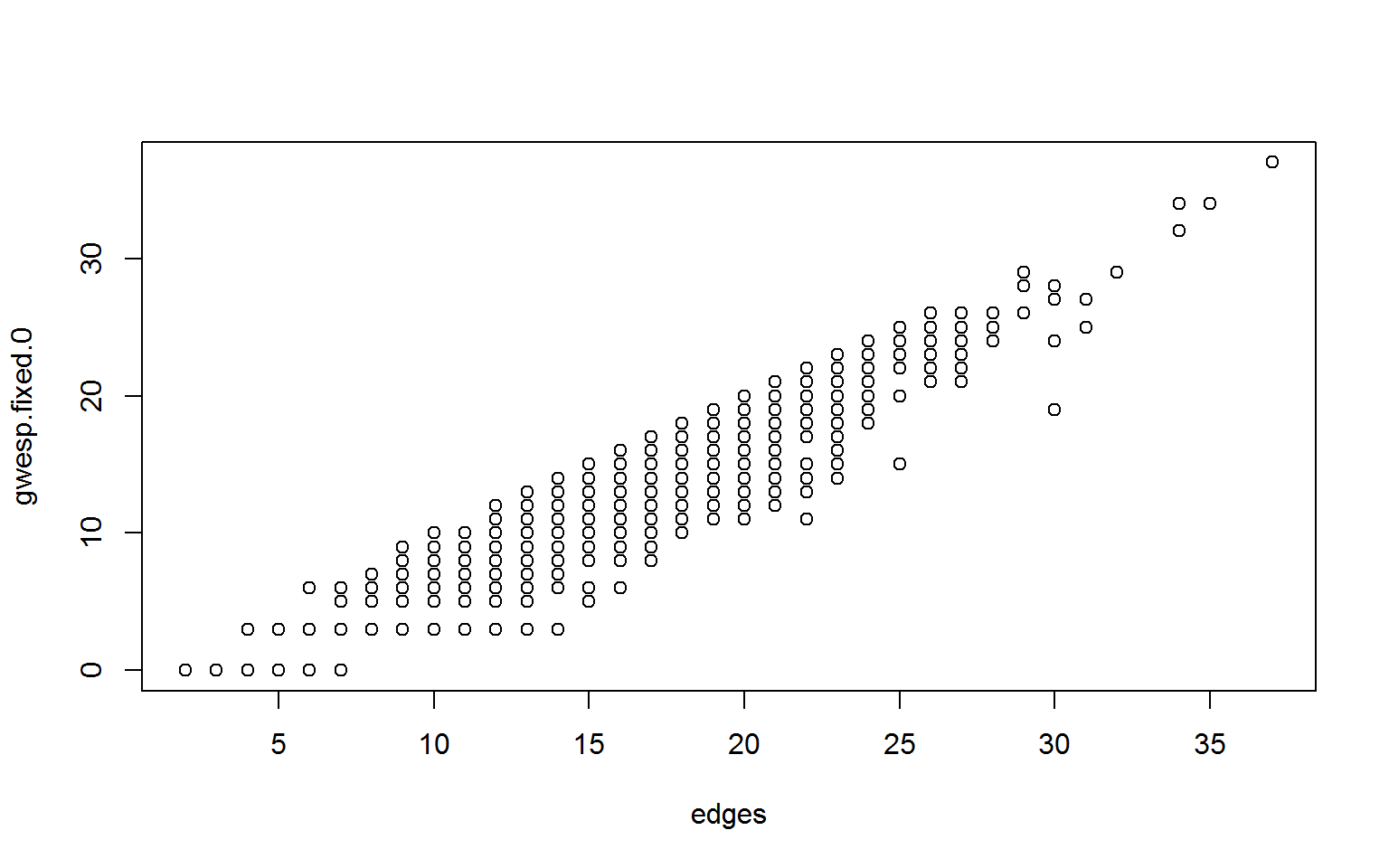We should also check to make sure that our mean duration is what we expect (10 time steps). This requires knowing an additional function: as.data.frame, which, when applied to an object of class networkDynamic, generates a timed edgelist. Although right-censoring is present for some edges in our simulation, with a mean duration of 10 time steps and a simulation 1000 time steps long, its effect on our observed mean duration should be small.

stergm.sim.1.df <- as.data.frame(stergm.sim.1)
names(stergm.sim.1.df)
 "onset"             "terminus"          "tail"
 "head"              "onset.censored"    "terminus.censored"
 "duration"          "edge.id"          
stergm.sim.1.df[1,]
  onset terminus tail head onset.censored terminus.censored duration
1     0        6    3    5           TRUE             FALSE        6
edge.id
1       1
mean(stergm.sim.1.df$duration)  10.09 The information on when an edge is active and when it is inactive is stored within our network object as the edge attribute active. Vertices, too, are capable of becoming active and inactive within networkDynamic, and this information is stored as a vertex attribute. Most of the time, users should access this information indirectly, through functions like network.extract or as.data.frame. Additional functions to query or set activity include is.active, activate.vertex, deactivate.vertex, activate.edge, and deactivate.edge, all documented in help(package="networkDynamic"). Note that networkDynamic stores spells in the form [onset,terminus), meaning that the spell is inclusive of the onset and exclusive of the terminus. So a spell of 3,7 means the edge begins at time point 3 and ends just before time point 7. networkDynamic can handle continuous-time spell information. However, since STERGMs are discrete-time with integer steps, what this means for STERGM is that the edge is inactive up through time step 2; active at time steps 3, 4, 5, and 6; and inactive again at time step 7 and on. Its duration is thus 4 time steps. ## 9. Visualizing dynamic networks using ndtv The Network Dynamic Temporal Visualization (ndtv) package provides tools for visualizing changes in network structure and attributes over time. ndtv has its own tutorial at Sunbelt, with materials at https://statnet.csde.washington.edu/trac/wiki/Sunbelt2014#WorkshopMaterials A quick taste of its functionality is here: install.packages('ndtv') library(ndtv) stergm.sim.1a <- simulate.stergm(stergm.fit.1, nsim=1, time.slices = 100) slice.par=list(start = 0, end = 25, interval=1, aggregate.dur=1, rule="any") compute.animation(stergm.sim.1a, slice.par = slice.par) render.par=list(tween.frames=5,show.time=T, show.stats="~edges+gwesp(0,fixed=T)") wealthsize <- log(get.vertex.attribute(flobusiness, "wealth")) * 2/3 render.animation(stergm.sim.1a,render.par=render.par, edge.col="darkgray",displaylabels=T, label.cex=.8,label.col="blue", vertex.cex=wealthsize) x11() ani.replay() ## 10. Independence within and across time steps STERGMs assume that the formation and dissolution processes are indepedent of each other within the same time step. This does not necessarily mean that they will be independent across time. In fact, for any dyadic dependent model, they will not. To see this point, think of a romantic relationship example with:  formation = ~edges+degree(2:10) dissolution = ~edges with increasingly negative parameters on the degree terms. What this means is that there is some underlying tendency for relational formation to occur, which is considerably reduced with each pre-existing tie that the two actors involved are already in. In other words, there is a strong prohibition against being in multiple simultaneous romantic relationships. However, dissolution is fully independent—all existing relationships have the same underlying dissolution probability at every time step. (The latter assumption is probably unrealistic; in practice, if someone just acquired a second partner, their first is likely to be at increased risk of dissoving their relation. We ignore this now for simplicity). Imagine that Chris and Pat are in a relationship at time t. During the time period between t and t+1, whether they break up does not depend on when either of them acquires a new partner, and vice versa. Let us assume that they do not break up during this time. Now, during the time period between t+1 and t+2, whether or not they break up is dependent on the state of the network at time t+1, and that depends on whether either of them they acquired new partners between t and t+1. The simple implication of this is that in this framework, formation and dissolution can be dependent, but that dependence occurs in subsequent time steps, not simultaneously. Note that a time step here is abritrary, and left to the user to define. One reason to select a smaller time interval is that it makes this assumption more justifiable. With a time step of 1 month, then if I start a new relationship today, the earliest I can break up with my first partner as a direct result of that new partnership is in one month. If my time step is a day, then it is in 1 day; the latter is likely much more reasonable. The tradeoff is that a shorter time interval means longer computation time for both model estimation and simulation, as will be seen below. You will see throughout this talk that there are multiple positives and negatives to having a short time step and having a long time step. At the limit, this can in practice approximate a continuous-time model—the only issue is computational limitations. ## 11. Example 3: Approximation with long durations For the type of model we saw in Example 2 (with a known dissolution model that contains a subset of terms from the formation model), it can be shown that a good set of starting values for the estimation of the formation model are as follows: 1. fit the terms in the formation model as a static ERGM on the cross-sectional network; and 2. subtract the values of the dissolution parameters from the corresponding values in the cross-sectional model. The result is a vector of parameter values that form a reasonable place to start the MCMC chain for the estimation of the formation model. This is in fact exactly what the stergm estimation code does by default for this type of model. When mean relational duration is very long, this approximation is so good that it may not be necessary to run a STERGM estimation at all. Especially if your purpose is mainly for simulation, the approximation may be all you need. This is a very useful finding, since models with long mean duration are precisely the ones that are the slowest and most difficult to fit using EGMME. That’s because, with long durations, very few ties will change between one time step and another, giving the fitting algorithm very little information on which to perform the estimation. One way to frame this is to think of the classic approximation from epidemiology. Under some circumstances: $$prevalence \approx incidence \times duration$$ which can be re-written as $$incidence \approx prevalence \div duration$$ The same relationship that holds for levels of infection also holds for levels of relationships under some condtions (in this case, long relationships). The dissolution/persistence model gives us log-odds that map onto duration. Fitting the cross-sectional ergm gives us log-odds for prevalence. We want to estimate incidence, so we subtract the two, rather than divide, since we are working on a log scale. Of course, in order to be able to take advantage of this method, it is necessary for the terms in your dissolution model to be a subset of the terms in your formation model. To illustrate, let us reconsider Example 2, with a mean relational duration of 100 time steps. theta.diss.100 <- log(99) First, we treat the formation process as if it were a stand-alone cross-sectional model, and estimate it using a standard cross-sectional ERGM. We did, in fact, fit this cross-sectional model earlier: summary(fit1)  ========================== Summary of model fit ========================== Formula: flobusiness ~ edges + gwesp(0, fixed = T) Iterations: 20 Monte Carlo MLE Results: Estimate Std. Error MCMC % p-value edges -3.354 0.606 0 <1e-04 *** gwesp.fixed.0 1.551 0.577 0 0.0082 ** --- Signif. codes: 0 '***' 0.001 '**' 0.01 '*' 0.05 '.' 0.1 ' ' 1 Null Deviance: 166.4 on 120 degrees of freedom Residual Deviance: 78.1 on 118 degrees of freedom AIC: 82.1 BIC: 87.7 (Smaller is better.)  theta.form <- fit1$coef
theta.form
        edges gwesp.fixed.0
-3.354         1.551 

Then, we subtract the values of the dissolution $$\theta$$ from each of the corresponding values in the formation model. In this example, the dissolution model contains only an edges term, so this coefficient should be subtracted from the starting value for the edges term in the formation model.

theta.form <- theta.form - theta.diss.100

How well does this approximation do in capturing our desired dynamic network properties? First, we can simulate from it:

stergm.sim.2 <- simulate(flobusiness, formation=~edges+gwesp(0,fixed=T),
dissolution=~edges, monitor="all",
coef.form=theta.form, coef.diss=theta.diss.100,
time.slices=50000)

Then check the results in terms of cross-sectional network structure and mean relational duration.

summary(flobusiness~edges+gwesp(0,fixed=T))
        edges gwesp.fixed.0
15            12 
colMeans(attributes(stergm.sim.2)$stats)  edges gwesp.fixed.0 15.31 12.13  stergm.sim.dm.2 <- as.data.frame(stergm.sim.2) mean(stergm.sim.dm.2$duration)
 99.64
plot(attributes(stergm.sim.2)$stats)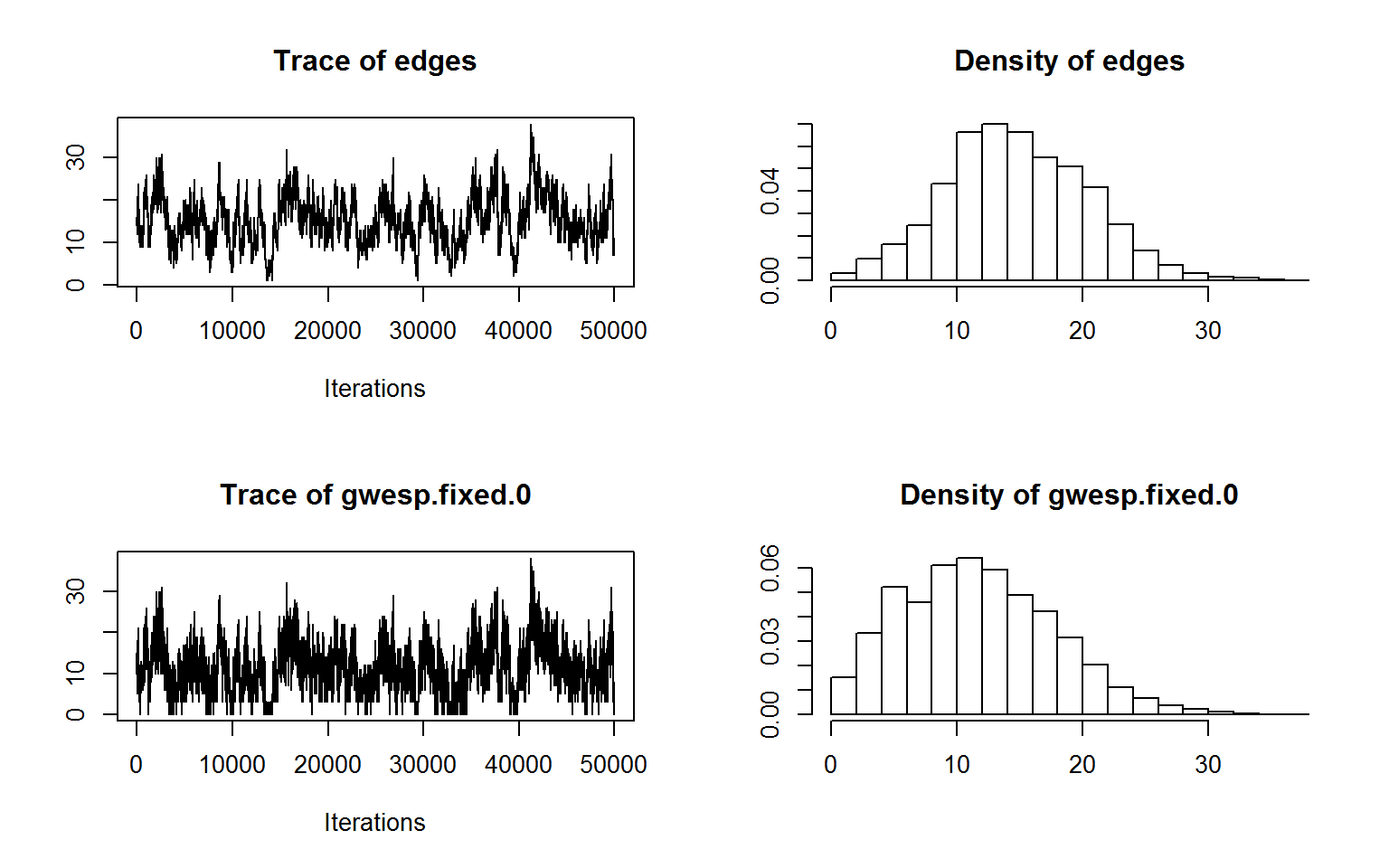## 12. Example 4: Simulation driven by egocentric data In many cases, people’s primary interest in using dynamic networks is to simulate some diffusion process on one or more networks with similar features. Increasingly, our knowledge about those features come in the form of egocentrically sampled data, not from the traditional network census in a bounded population. Both ergm and stergm have methods for handling these situations. For example, imagine that you want to model HIV transmission among a population of gay men in steady partnerships. 50% of the men are White and 50% are Black. You collect egocentric partnership data from a vaguely random sample of these men. Your data say: • There are no significant differences in the distribution of momentary degree (the number of ongoing partnerships at one point in time) reported by White vs. Black men. The mean is 0.90, and the overall distribution is: • 36% degree 0 • 46% degree 1 • 18% degree 2+ • 83.3% (i.e. 5/6) of relationships are racially homogeneous We also have data (from these same men, or elsewhere) that tell us that the mean duration for a racially homogenous relationship is 10 months, while for a racially mixed one it is 20 months. Perhaps this is because the social pressure against cross-race ties makes it such that those who are willing to enter them are a select group more committed to their relationships. Before we model the disease transmission, we need a dynamic network that possesses each of these features to simulate it on. Our first step is to create a 500-node undirected network, and assign the first 250 nodes to race 0 and the second to race 1. The choice of 500 nodes is arbitary. msm.net <- network.initialize(500, directed=F) msm.net %v% 'race' <- c(rep(0,250),rep(1,250)) msm.net  Network attributes: vertices = 500 directed = FALSE hyper = FALSE loops = FALSE multiple = FALSE bipartite = FALSE total edges= 0 missing edges= 0 non-missing edges= 0 Vertex attribute names: race vertex.names No edge attributes ERGM and STERGM have functionality that allow us to simply state what the target statistics are that we want to match; we do not actually need to generate a network that has them. One way that we can specify the formation formula and target statistics is: msm.form.formula <- ~edges+nodematch('race')+degree(0)+ concurrent msm.target.stats <- c(225,187,180,90) How did we get those values? Why don’t we specify degree(1) as well? Now let us turn to dissolution. We are back to the case where we can solve these explicitly, although this is complicated slightly by the fact that our dissolution probabilities differ by the race composition of the members. One dissolution formula for representing this is: msm.diss.formula <- ~offset(edges)+offset(nodematch("race")) These two model statistics means that there will be two model coefficients. Let us call them $$\theta_1$$ and $$\theta_2$$ for the edges and nodematch terms, respectively. Let us also refer to the change statistics for actor pair $$i,j$$ for each of these as $$\delta(g_1(y))_{ij}$$ and $$\delta(g_2(y))_{ij}$$, respectively. Thus the log-odds expression for dissolution that we saw earlier would here be expressed as: $$\ln{\frac{P(Y_{ij,t+1}=1 \mid Y_{ij,t}=1)}{P(Y_{ij,t+1}=0\mid Y_{ij,t}=1)}} = \theta_1\delta(g_1(y))_{ij}+\theta_2\delta(g_2(y))_{ij}$$ which, in words, means that the conditional log-odds of a tie persisting equals $$\theta_1$$ times the number of ties it involves, plus $$\theta_2$$ times the number of race-homophilous ties it involves. Note, then, that $$\delta(g_1(y))_{ij}$$ would equal 1 for all actor pairs, while $$\delta(g_2(y))_{ij}$$ would equal 1 for race homophilous pairs and 0 for others. That means that the log-odds of tie persistence will equal $$\theta_1$$ for mixed-race couples and $$\theta_1 + \theta_2$$ for race-homophilous couples. This suggests that we should be able to calculate $$\theta_1$$ directly, and subsequently calculate $$\theta_2$$. Following the logic we saw in Example 2, we can see that: $$\theta_1 = \ln{(d_{mixed}-1)}$$ and therefore $$\theta_1 = \ln{(20-1)} = \ln{19} = 2.944$$ Furthermore, $$\theta_1 + \theta_2 = \ln{(d_{homoph}-1)}$$ and therefore $$\theta_2 = \ln({d_{homoph}-1)}-\theta_1 = \ln{(10-1)} - 2.944 = -0.747$$. So, we have: msm.theta.diss <- c(2.944, -0.747)  We add in one additional control parameter—SA.init.gain—giving it a small value (the default is 0.1). As the help page for control.stergm sagely advises, ‘’If the process initially goes crazy beyond recovery, lower this value.’’ This slows down estimation, but also makes it more stable. From trial and error, we know that this model, fit to this relatively large network, does better with this smaller value. Putting it all together (including the syntax to control the window for real-time monitoring) gives us: X11() msm.fit <- stergm(msm.net, formation= msm.form.formula, dissolution= msm.diss.formula, targets="formation", target.stats= msm.target.stats, offset.coef.diss = msm.theta.diss, estimate = "EGMME", control=control.stergm(SA.plot.progress=TRUE, SA.init.gain=0.005) ) dev.off() Iteration 1 of at most 20: ====== Lots of output snipped. ====== ======== Phase 3: Simulate from the fit and estimate standard errors. ======== Let’s first check to make sure it fit well: mcmc.diagnostics(msm.fit)  ========================== EGMME diagnostics ========================== Sample statistics summary: Iterations = 13:3262 Thinning interval = 1 Number of chains = 1 Sample size per chain = 3250 1. Empirical mean and standard deviation for each variable, plus standard error of the mean: Mean SD Naive SE Time-series SE edges 1.434 14.4 0.252 1.082 nodematch.race 0.981 13.6 0.238 1.015 degree0 -1.154 13.5 0.236 0.753 concurrent 0.914 10.9 0.192 0.803 2. Quantiles for each variable: 2.5% 25% 50% 75% 97.5% edges -26.0 -8 1 11 29 nodematch.race -25.0 -8 1 10 28 degree0 -27.0 -11 -2 8 26 concurrent -19.8 -7 1 8 23 Are sample statistics significantly different from observed? edges nodematch.race degree0 concurrent Overall (Chi^2) diff. 1.4345 0.9806 -1.1545 0.9135 NA test stat. 1.3252 0.9663 -1.5339 1.1379 3.0155 P-val. 0.1851 0.3339 0.1251 0.2551 0.5552 Sample statistics cross-correlations: edges nodematch.race degree0 concurrent edges 1.0000 0.9080 -0.8524 0.8781 nodematch.race 0.9080 1.0000 -0.7772 0.7907 degree0 -0.8524 -0.7772 1.0000 -0.5859 concurrent 0.8781 0.7907 -0.5859 1.0000 Sample statistics auto-correlation: Chain 1 edges nodematch.race degree0 concurrent Lag 0 1.0000 1.0000 1.0000 1.0000 Lag 1 0.8971 0.8955 0.8208 0.8917 Lag 2 0.8004 0.7977 0.6687 0.7967 Lag 3 0.7147 0.7117 0.5489 0.7182 Lag 4 0.6349 0.6323 0.4474 0.6495 Lag 5 0.5660 0.5628 0.3683 0.5877 Sample statistics burn-in diagnostic (Geweke): Chain 1 Fraction in 1st window = 0.1 Fraction in 2nd window = 0.5 edges nodematch.race degree0 concurrent 0.5908 0.5128 -0.2263 0.6371 P-values (lower = worse): edges nodematch.race degree0 concurrent 0.5546 0.6081 0.8209 0.5241  Loading required package: latticeExtra Loading required package: RColorBrewer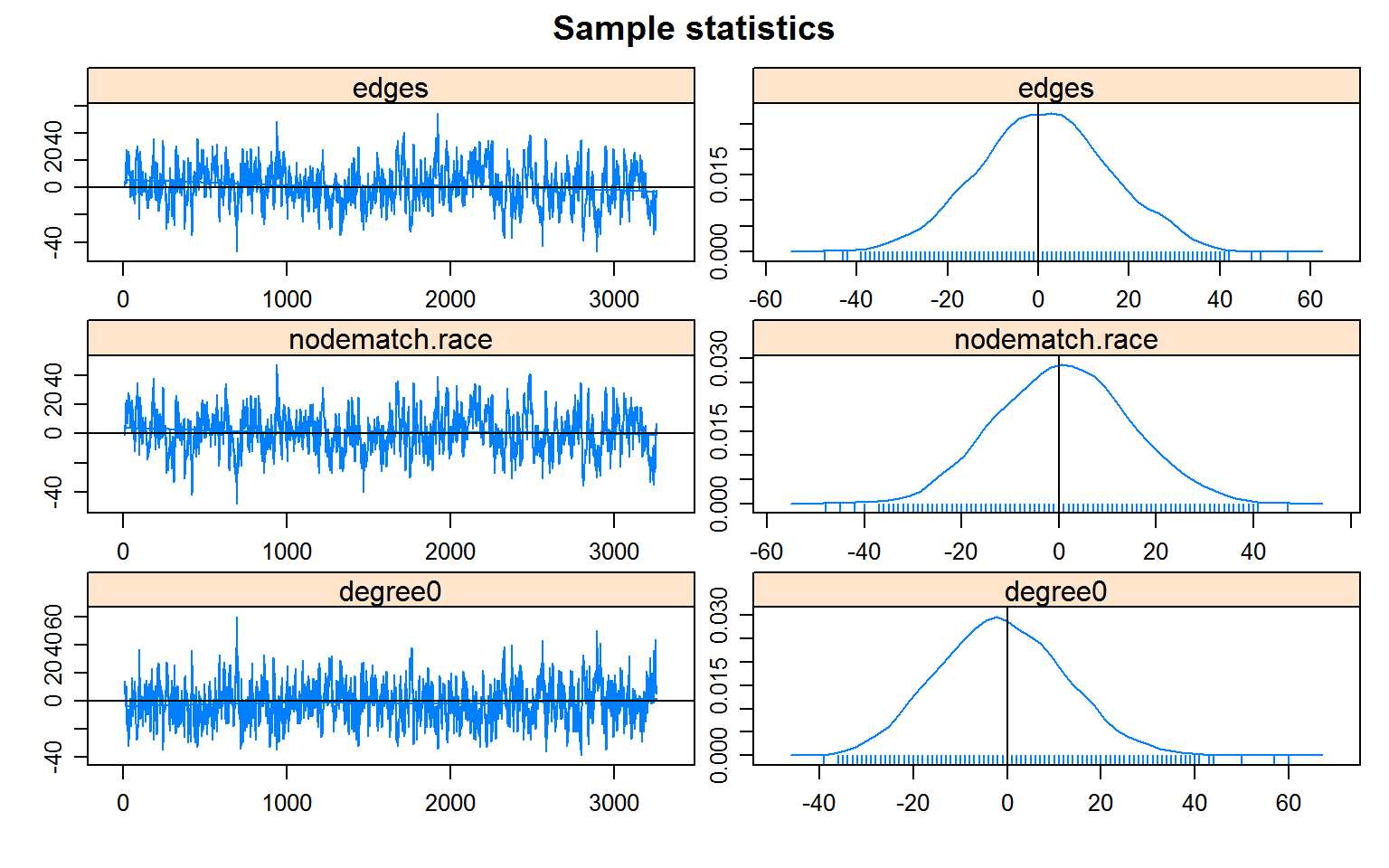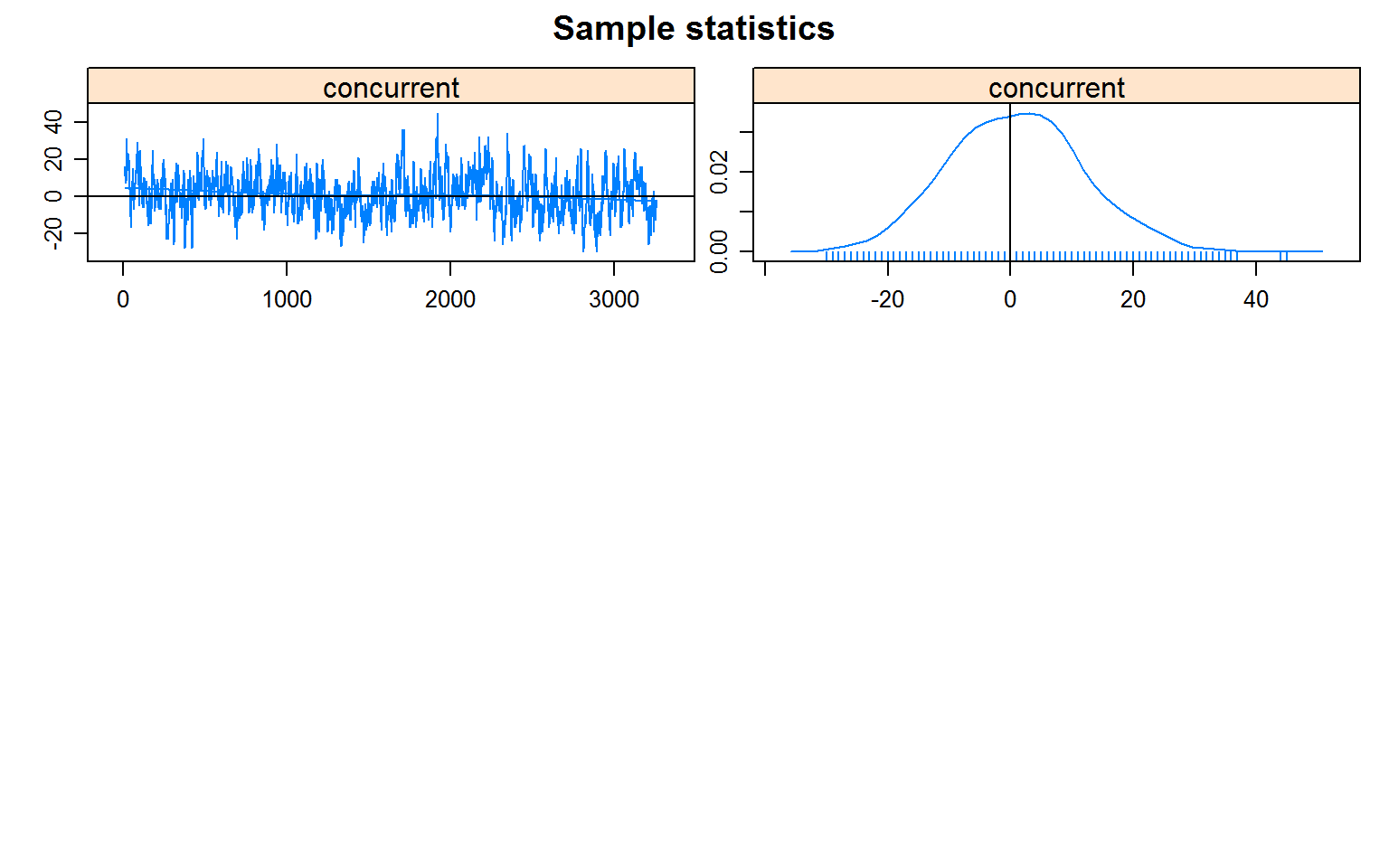and see what the results tell us: summary(msm.fit)  ============================== Summary of formation model fit ============================== Formula: ~edges + nodematch("race") + degree(0) + concurrent Iterations: NA Equilibrium Generalized Method of Moments Results: Estimate Std. Error MCMC % p-value edges -9.955 0.570 0 <1e-04 *** nodematch.race 2.289 0.406 0 <1e-04 *** degree0 -0.200 0.168 0 0.2335 concurrent -0.824 0.263 0 0.0017 ** --- Signif. codes: 0 '***' 0.001 '**' 0.01 '*' 0.05 '.' 0.1 ' ' 1 ================================ Summary of dissolution model fit ================================ Formula: ~offset(edges) + offset(nodematch("race")) Iterations: NA Equilibrium Generalized Method of Moments Results: Estimate Std. Error MCMC % p-value edges 2.944 NA NA NA nodematch.race -0.747 NA NA NA The following terms are fixed by offset and are not estimated: edges nodematch.race  One should not over-interpret the standard errors and p-values in this case. Remember, the size of the population that we built to fit this model was arbitrary (although it could have been constructed to match the source data). Our main goal in fitting the model is to be able to simulate a dynamic network with both desired cross-sectional and durational features maintained stochastically over time. Now, to do that, we simulate a dynamic network: msm.sim <- simulate(msm.fit,time.slices=1000) and compare the outputs to what we expect, in terms of cross-sectional structure: colMeans(attributes(msm.sim)$stats)
         edges nodematch.race        degree0     concurrent
227.18         188.43         178.71          91.57 
msm.target.stats
 225 187 180  90

And relationship length:

race <- msm.net %v% 'race'
msm.sim.dm <- as.data.frame(msm.sim)
names(msm.sim.dm)
 "onset"             "terminus"          "tail"
 "head"              "onset.censored"    "terminus.censored"
 "duration"          "edge.id"          
mean(msm.sim.dm$duration[race[msm.sim.dm$tail] ==  race[msm.sim.dm$head]])  10.01 mean(msm.sim.dm$duration[race[msm.sim.dm$tail] != race[msm.sim.dm$head]])
 19.59

Don’t run this in real-time during the tutorial, as it is slow, but if you wished to visualize this dynamic network, the following code would do so:

slice.par=list(start = 0, end = 100, interval=1, aggregate.dur=1, rule="any")
compute.animation(msm.sim, slice.par = slice.par)

render.par=list(tween.frames=5,show.time=T)
render.animation(msm.sim,render.par=render.par,
edge.col="darkgray", displaylabels=F, vertex.col="race")
x11()
ani.replay()

## 13. Additional functionality

Both the stergm functions and the networkDynamic package have additional functionality, which you can learn about and explore through the use of R’s many help features.

If you begin to use them in depth, you will likely have further questions. If so, we encourage you to join the statnet users’ group (http://csde.washington.edu/statnet/statnet_users_group.shtml), where you can then post your questions (and possibly answer others). You may also encounter bugs; please use the same place to report them.